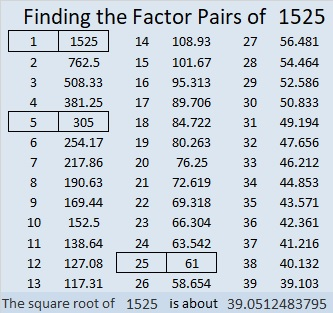# 1525 Challenge Puzzle

Contents

### Today’s puzzle:

There is plenty to challenge you in solving this puzzle, but there are also quite a few very helpful clues. Just remember to use logic and have fun with it!Print the puzzles or type the solution in this excel file: 12 Factors 1511-1525

### Factors of 1525:

• 1525 is a composite number.
• Prime factorization: 1525 = 5 × 5 × 61, which can be written 1525 = 5² × 61.
• 1525 has at least one exponent greater than 1 in its prime factorization so √1525 can be simplified. Taking the factor pair from the factor pair table below with the largest square number factor, we get √1525 = (√25)(√61) = 5√61.
• The exponents in the prime factorization are 2 and 1. Adding one to each exponent and multiplying we get (2 + 1)(1 + 1) = 3 × 2 = 6. Therefore 1525 has exactly 6 factors.
• The factors of 1525 are outlined with their factor pair partners in the graphic below.### More about the Number 1525:

1525 is the sum of two squares in two different ways:
39² + 2² = 1525
38² + 9² = 1525

1525 is the hypotenuse of a Pythagorean triple in SEVEN different ways!
156-1517-1525, calculated from 2(39)( 2), 39² – 2², 39² + 2²,
275-1500-1525, which is 25 times (11-60-61),
427-1464-1525, which is (7-24-25) times 61,
680-1365-1525, which is 5 times (136-273-305)
684-1363-1525, calculated from 2(38)( 9), 38² – 9², 38² + 9²,
915-1220-1525, which is (3-4-5) times 305, and
1035-1120-1525, which is 5 times (207-224-305).

1525 is also the difference of two squares in two different ways:
763² – 762² = 1525,
155² – 150² = 1525, and
43² – 18² = 1525.

1525 is the 25th heptagonal number because
(5(25²)-3(25))/2 = 1525.
That means you can make a 7-sided figure out of 1525 dots where two of the sides are shared with all the previous heptagons.

This site uses Akismet to reduce spam. Learn how your comment data is processed.